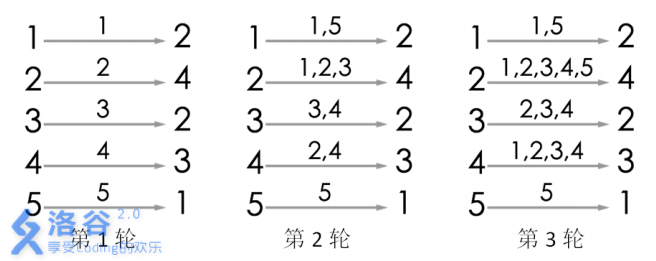## 输出格式

11个整数，表示游戏一共可以进行多少轮。

## 输入输出样例

5
2 4 2 3 1

3

## 说明/提示#include<iostream>
#include<algorithm>
using namespace std;
const int maxn = 2e5+5;
int father[maxn];
int dis[maxn];
int minn;
void init()
{
for(int i=1;i<maxn;++i)
{
father[i]=i;
dis[i]=0;
}
}
int find(int x)
{
if(x != father[x])
{
int temp = father[x];
father[x] = find(father[x]);
dis[x] += dis[temp];
}
//cout<<"test"<<endl;
return father[x];
}

void merge(int x,int y)
{
int p = find(x);
int q = find(y);
if(p!=q)
{
father[p]=q;
dis[x] = dis[x]+2;
//dis[p]=1;
}else
{
minn = min(minn,dis[x]+dis[y]+1);
}
}

int main()
{
int n,t;
while(cin>>n)
{
minn = 1<<30;
init();
for(int i=1;i<=n;++i)
{
cin>>t;
merge(i,t);
}
cout<<minn<<endl;
}
return 0;
}
Last modification：January 12th, 2020 at 01:52 am